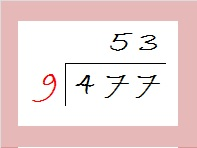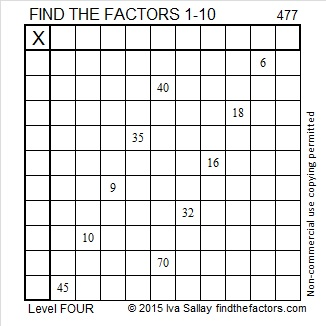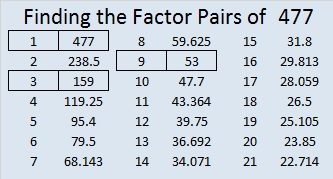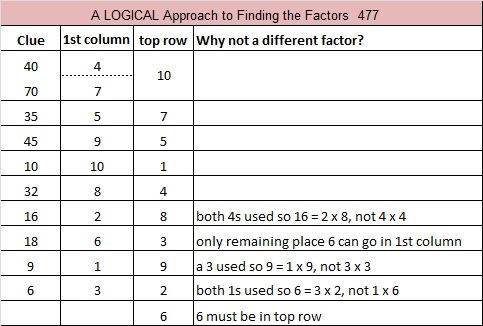# 477 and Level 4

4 + 7 + 7 = 18, a multiple of 9, so 477 can be evenly divided by 9, and 477 is the third number in a row whose square root can be simplified.To find the square root we only need to make a one-layer cake before we take the square root of everything on the outside of the cake: √477 = (√9)(√53) = 3√53Print the puzzles or type the solution on this excel file: 10 Factors 2015-04-27

—————————————————————————————————

• 477 is a composite number.
• Prime factorization: 477 = 3 x 3 x 53, which can be written 477 = (3^2) x 53
• The exponents in the prime factorization are 2 and 1. Adding one to each and multiplying we get (2 + 1)(1 + 1) = 3 x 2  = 6. Therefore 477 has exactly 6 factors.
• Factors of 477: 1, 3, 9, 53, 159, 477
• Factor pairs: 477 = 1 x 477, 3 x 159, or 9 x 53
• Taking the factor pair with the largest square number factor, we get √477 = (√9)(√53) = 3√53 ≈ 21.84033—————————————————————————————————This site uses Akismet to reduce spam. Learn how your comment data is processed.